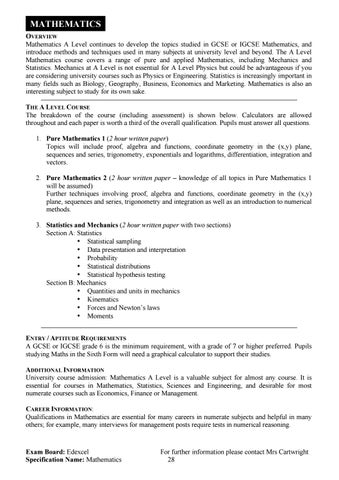MATHEMATICS

OVERVIEW Mathematics A Level continues to develop the topics studied in GCSE or IGCSE Mathematics, and introduce methods and techniques used in many subjects at university level and beyond. The A Level Mathematics course covers a range of pure and applied Mathematics, including Mechanics and Statistics. Mechanics at A Level is not essential for A Level Physics but could be advantageous if you are considering university courses such as Physics or Engineering. Statistics is increasingly important in many fields such as Biology, Geography, Business, Economics and Marketing. Mathematics is also an interesting subject to study for its own sake. THE A LEVEL COURSE The breakdown of the course (including assessment) is shown below. Calculators are allowed throughout and each paper is worth a third of the overall qualification. Pupils must answer all questions. 1. Pure Mathematics 1 (2 hour written paper) Topics will include proof, algebra and functions, coordinate geometry in the (x,y) plane, sequences and series, trigonometry, exponentials and logarithms, differentiation, integration and vectors. 2. Pure Mathematics 2 (2 hour written paper – knowledge of all topics in Pure Mathematics 1 will be assumed) Further techniques involving proof, algebra and functions, coordinate geometry in the (x,y) plane, sequences and series, trigonometry and integration as well as an introduction to numerical methods. 3. Statistics and Mechanics (2 hour written paper with two sections) Section A: Statistics • Statistical sampling • Data presentation and interpretation • Probability • Statistical distributions • Statistical hypothesis testing Section B: Mechanics • Quantities and units in mechanics • Kinematics • Forces and Newton’s laws • Moments ENTRY / APTITUDE REQUIREMENTS A GCSE or IGCSE grade 6 is the minimum requirement, with a grade of 7 or higher preferred. Pupils studying Maths in the Sixth Form will need a graphical calculator to support their studies. ADDITIONAL INFORMATION University course admission: Mathematics A Level is a valuable subject for almost any course. It is essential for courses in Mathematics, Statistics, Sciences and Engineering, and desirable for most numerate courses such as Economics, Finance or Management. CAREER INFORMATION: Qualifications in Mathematics are essential for many careers in numerate subjects and helpful in many others; for example, many interviews for management posts require tests in numerical reasoning.

Exam Board: Edexcel Specification Name: Mathematics